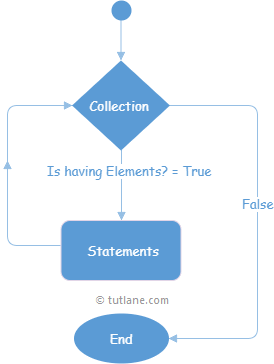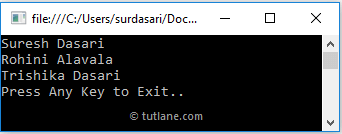# Visual Basic (VB) For Each Loop

In Visual Basic, For Each loop is useful to loop through items in an array or collection object to repeatedly execute the block of statements.

In Visual Basic For Each loop will work with the collection objects such as an array, list, etc., to execute the block of statements for each element in the array or collection.

After iterating through each element in a collection, control will transfer to the next statement of the following For Each block.

In Visual Basic, we can use Exit, Continue statements within For Each loop to exit or continue to the next iteration of the loop based on our requirements.

## Visual Basic For Each Loop Syntax

Following is the syntax of defining the For Each loop in Visual Basic programming language.

For Each var_name As [Data_Type] In Collection_Object
// Statements to Execute
Next

If you observe the above syntax, we defined For Each loop with a collection object and required variable name to access elements from the collection object.

Here, Data_Type is a built-in data type or custom class type, and var_name is a variable name to access elements from the collection object (Collection_Object) to use in the body of For Each loop.

## Visual Basic Foreach Loop Flow Chart

Following is the pictorial representation of For Each loop process flow diagram in Visual Basic programming language.Now, we will see how to use For Each loop in a visual basic programming language with examples.

## Visual Basic Foreach Loop with Array Example

Following is the example of using For Each loop in a visual basic programming language to iterate or loop through array elements.

Module Module1
Sub Main()
Dim names As String() = New String(2) {"Suresh Dasari", "Rohini Alavala", "Trishika Dasari"}
For Each name As String In names
Console.WriteLine(name)
Next
Console.WriteLine("Press Enter Key to Exit..")
End Sub
End Module

If you observe the above example, we created a string array object “names”. We looped through each element of the array object using For Each loop and assigning array elements to the string variable “name”.

To know more about arrays in a visual basic programming language, check Visual Basic arrays with examples.

When we execute the above Visual Basic program, we will get the result as shown below.If you observe the above result, we iterated through each array element and printed those values on the console window based on our requirements.

## Visual Basic Foreach Loop with List Example

Same as For Each with arrays, we can use For Each loop with list object to process each element in the list object, but inside For Each loop, it won’t allow us to modify (add or delete) the list object items.

Following is the example of using For Each loop in a visual basic programming language to iterate or loop through list elements.

Module Module1
Sub Main()
Dim names As List(Of String) = New List(Of String)() From {
"Suresh Dasari",
"Rohini Alavala",
"Trishika Dasari"
}
For Each name As String In names
Console.WriteLine(name)
Next
Console.WriteLine("Press Enter Key to Exit..")
End Sub
End Module

If you observe the above example, we created and added the string elements to the names list object. Here, we used For Each to loop through the items in the list to print it on the console window.

When we execute the above visual basic program, we will get the result below.This is how we can use For Each loop in a visual basic programming language to loop through each element in the array or collection objects based on our requirements.